# Square root + right triangle - math problems

#### Number of problems found: 123

• Right angled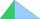From the right triangle with legs 12 cm and 20 cm we built a square with the same content as the triangle. How long will be side of the square?
• Triangle ABCRight triangle ABC with right angle at the C, |BC|=18, |AB|=33. Calculate the height of the triangle hAB to the side AB.
• Is right?Is a triangle with sides 51, 56, and 77 right triangle?
• Without Euclid lawsRight triangle ABC with right angle at the C has a=14 and hypotenuse c=26. Calculate the height h of this triangle without the use of Euclidean laws.
• Euklid4The legs of a right triangle have dimensions 244 m and 246 m. Calculate the length of the hypotenuse and the height of this right triangle.
• Leg and heightSolve right triangle with height v = 9.6 m and shorter cathetus b = 17.3 m.
• Isosceles right triangleCalculate the area of an isosceles right triangle whose perimeter is 252 cm.
• Right triangleLegs of the right triangle are in the ratio a:b = 2:8. The hypotenuse has a length of 87 cm. Calculate the perimeter and area of the triangle.
• Square2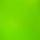Side of the square is a = 6.2 cm, how long is its diagonal?
• Square 2Points D[10,-8] and B[4,5] are opposed vertices of the square ABCD. Calculate area of the square ABCD.
• Right isoscelesCalculate area of the isosceles right triangle which perimeter is 26 cm.
• Height UTHow long is height in the equilateral triangle with a side b = 43?
• Square and circlesSquare with sides 83 cm is circumscribed and inscribed with circles. Determine the radiuses of both circles.
• Is right-angled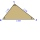Can a triangle with the sides of sqrt 3, sqrt 5 and sqrt 8 (√3, √5 and √8) be a right triangle?
• Right triangle - leg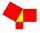Calculate to the nearest tenth cm length of leg in right-angled triangle with hypotenuse length 9 cm and 7 cm long leg.
• OPT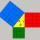What is the perimeter of a right triangle with the legs 14 cm and 21 cm long?
• Area of RTIn the right triangle has orthogonal projections of legs to the hypotenuse lengths 15 cm and 9 cm. Determine the area of ​​this triangle.
• Square diagonalCalculate the length of the square diagonal if the perimeter is 172 cm.
• SquareRectangular square has side lengths 183 and 244 meters. How many meters will measure the path that leads straight diagonally from one corner to the other?
• HypotenuseCalculate the length of the hypotenuse of a right triangle with a catheti 71 cm and 49 cm long.

Do you have an interesting mathematical word problem that you can't solve it? Submit a math problem, and we can try to solve it.

We will send a solution to your e-mail address. Solved examples are also published here. Please enter the e-mail correctly and check whether you don't have a full mailbox.

Please do not submit problems from current active competitions such as Mathematical Olympiad, correspondence seminars etc...

See also our right triangle calculator. Square root - math problems. Right triangle Problems.